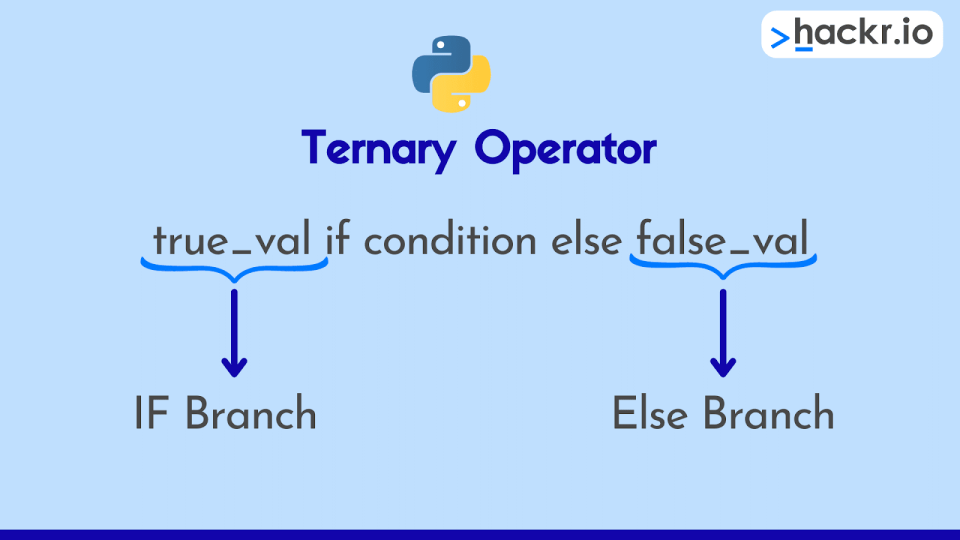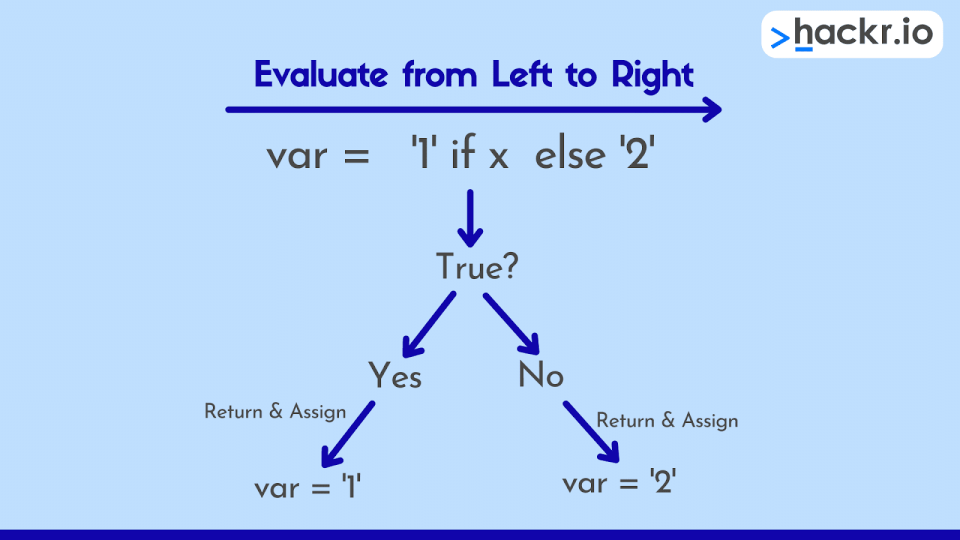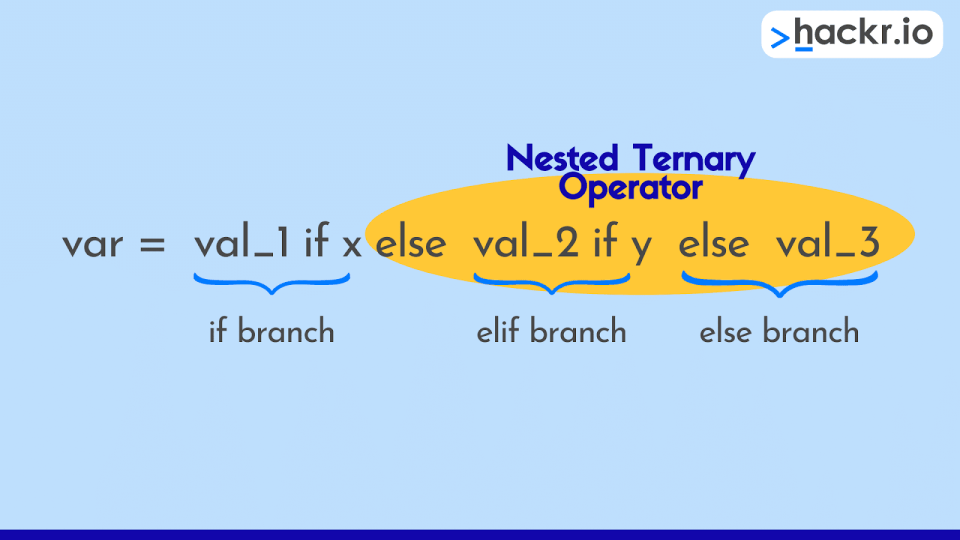Are you looking to get a discount on popular programming courses? Then click here. View offers

## Code, Programming and Python

Disclosure: Hackr.io is supported by its audience. When you purchase through links on our site, we may earn an affiliate commission.

# Python Ternary Operator: How and Why You Should Use It

Posted in Code, Programming, PythonWriting concise, practical, organized, and intelligible code should be a top priority for any Python developer. Enter, the Python ternary operator.

What is this? I hear you ask. Well, you can use the ternary operator to test a condition and then execute a conditional assignment with only one line of code. And why is this so great? Well, this means we can replace those ever-popular if-else statements with a quicker and more practical way to code conditional assignments.

Now don’t get me wrong, we still need if-else statements (check out our comprehensive guide and Python cheat sheet for the best way to use conditional statements). But, it’s always good practice to make your code more Pythonic when there’s a simpler way to get the same result, and that’s exactly what you get with the ternary operator.

## Why Use the Python Ternary Operator?

Ternary operators (also known as conditional operators) in Python perform evaluations and assignments after checking whether a given condition is true or false. This means that the ternary operator is a bit like a simplified, one-line if-else statement. Cool, right?

When used correctly, this operator reduces code size and improves readability.

### Ternary Operator Syntax

A ternary operator takes three operands:

• condition: A Boolean expression that evaluates to true or false
• true_value: A value or expression to be assigned if the condition evaluates to true
• false_value: A value or expression to be assigned if the condition evaluates to false

This is how it should look when we put it all together:

``var = true_value if [condition] else false_value``So, this means that the variable "var" on the left side of the assignment operator (=), will be assigned either:

• true_valueif the Boolean expression evaluates to true
• false_valueif the Boolean expression evaluates to false

## How to Use the Ternary Operator Instead of If-Else

To better understand how we replace an if-else statement with the ternary operator in Python, let’s write a simple program with each approach. In this first example, we’ll assume the user enters an integer number when prompted, and we’ll start with the familiar if-else.

### Program Using an If-Else Statement

``````given_age = input("Enter your age to check if you are old enough to watch this movie or not:")
movie_access = "Yes, you are old enough to watch this movie!" if int(given_age) >= 18 else "Sorry, you aren't old enough to watch this movie yet!"
print(f"The Result - {movie_access}")``````

Here’s what we get when the user enters 22 at the input prompt:

``````Enter your age to check if you are old enough to watch this movie or not: 22

The Result - Yes, you are old enough to watch this movie!``````

In this example, the if-else statement assigns “Yes, you are old enough to watch this movie!” to the movie_acess variable if the user enters an age that is greater than or equal to 18, otherwise, it assigns "Sorry, you aren't old enough to watch this movie yet!”. Finally, we use an f-string to print out the result to the screen.

Now, let's use the ternary operator syntax to make the program much more concise.

### Program Using a Ternary Operator

``````given_age = input('Enter your age to check if you are old enough to watch this movie or not:')
movie_access = ('Yes, you are old enough to watch this movie!' if int(given_age) >= 18 else 'Sorry, you are\'nt old enough to watch this movie yet')
print(f'The Result - {movie_access}')
``````

In this version of the program, the variable that receives the result of the conditional statement (movie_access) is to the left of the assignment operator (=).

The ternary operator evaluates the condition:

``if int(given_age) >= 18``

If the condition evaluates to true, the program assigns “Yes, you are old enough to watch this movie!” to movie_access, else it assigns “Sorry, you aren't old enough to watch this movie yet!”.

Let’s take a look at another example that uses the Python ternary operator and if-else to achieve the same goal. This time, we’ll write a simple Python code snippet to check whether a given integer is even or odd.

``````# Using Ternary Operator
given_int = 8
msg = "Even" if (given_int % 2) == 0 else "Odd"
print(msg)
# Using If-Else statement
msg = " "
if(given_int % 2) == 0:
msg = "Even"
else:
msg = "Odd"
print(msg)``````

In this program, we’ve used a ternary operator and an if-else statement block to determine whether the given integer produces a zero remainder when using modulo divide by 2 (if you’re unfamiliar with this operator, take a look at our cheat sheet). If the remainder is zero, we have an even number, otherwise, we have an odd number.

Right away, we can tell what the if-else statement will do after evaluating the condition. With the ternary operator snippet, we can see the following:

• "Even" is assigned to msg if the condition (given_int % 2 == 0) is true
• "Odd" is assigned to msg if the condition (given_int % 2 == 0) is false

And, as we’ve set the given integer to an even number, the program will print “Even” to the screen.

So, we can see again that a ternary conditional statement is a much cleaner and more concise way to achieve the same outcome as an if-else statement. We simply evaluate the ternary expression from left to right, then assign the return value for the true or false condition.## Key Considerations for Python Ternary Statements

The ternary operator is not always suitable to replace if-else in your code.

If for example, we have more than two conditional branches with an if-elif-else statement, we can’t replace this with a single ternary operator. The clue here is in the name, as ternary refers to the number ‘three’, meaning that it expects three operands. But, if we try to replace an if-elif-else statement with a ternary operator, we’ll have four operands to handle (or possibly more if we have many ‘elif’ branches).

So what do we do here? Well, we need to chain together (or nest) multiple ternary operators (we cover this in the FAQs), but sometimes this can get a little messy, and it may be cleaner to use if-elif-else if the code becomes difficult to read.Another thing to keep in mind is that we’re meant to use the ternary operator for conditional assignment. What does this mean? Well, we can’t use the ternary approach if we want to execute blocks of code after evaluating a conditional expression: in these situations, it’s best to stick with if-else.

But, we can use the ternary operator if we are assigning something to a variable after checking against a condition. See, it’s easy if we remember that we’re supposed to be assigning something to something else.

### Distinct Features of Python Ternary Statements

• Returns A or B depending on the Boolean result of the conditional expression (a < b)
• Compared with C-type languages(C/C++), it uses a different ordering of the provided arguments
• Conditional expressions have the lowest priority of all Python operations

## Conclusion

That's all. We did our best to fill you in on everything there is to know about the Python ternary operator. We also covered how to use ternary statements in your Python code, and we compared them to their close relatives, the ever-popular if-else statement.

If you’re a beginner that’s looking for an easy start to your Python programming career, check out our learning guide along with our up-to-date list of Python courses where you can easily enroll online.

Lastly, don’t forget that you can also check out our comprehensive Python cheat sheet which covers various topics, including Python basics, flow control, Modules in Python, functions, exception handling, lists, dictionaries, and data structures, sets, the itertools module, comprehensions, lambda functions, string formatting, the ternary conditional operator, and much more.

#### 1. What are Ternary Operators (including an example)?

Programmers like to use the concise ternary operator for conditional assignments instead of lengthy if-else statements.

The ternary operator takes three arguments:

• Firstly, the comparison argument
• Secondly, the value (or result of an expression) to assign if the comparison is true
• Thirdly, the value (or result of an expression) to assign if the comparison is false

#### Simple Ternary Operator Example:

``````m = 10
n = 20
o = m if (m < n) else n
print(o)``````

If we run this simple program, the "(m < n)" condition evaluates to true, which means that "m" is assigned to "o" and the print statement outputs the integer 10.

The conditional return values of "m" and "n" must not be whole statements, but rather simple values or the result of an expression.

#### 2. How Do You Write a 3-Condition Ternary Operator?

Say you have three conditions to check against and you want to use a ternary conditional statement. What do you do? The answer is actually pretty simple, chain together multiple ternary operators. The syntax below shows the general format for this:

``var = value_1 if [condition_1] else value_2 if [condition_2] else value_3``

If we break this syntax down, it is saying:

• If condition_1 is true, return value_1 and assign this to var
• Else, check if condition_2 is true. If it is, return value_2 and assign it to var
• If neither conditions are true, return value_3 and assign this to var

We’ve now created a one-line version of an if-elif-else statement using a chain of ternary operators.

The equivalent if-elif-else statement would be:

``````if condition_1:
var = value_1
elif condition_2:
var = value_2
else:
var = value_3
``````

One thing to consider before chaining together ternary statements is whether it makes the code more difficult to read. If so, then it’s probably not very Pythonic and you’d be better off with an if-elif-else statement.

#### 3. How Do You Code a Ternary Operator Statement?

We can easily write a ternary expression in Python if we follow the general form shown below:

``var_result = true_value if [condition] else false_value``

So, we start by choosing a condition to evaluate against. Then, we either return the true_value or the false_value and then we assign this to results_var

#### 4. Is the Ternary Operator Faster Than If-Else?

If we consider that a ternary operator is a single-line statement and an if-else statement is a block of code, then it makes sense that if-else will take longer to complete, which means that yes, the ternary operator is faster.

But, if we think about speed in terms of Big-O notation (if I’ve lost you here, don’t worry, head on over to our Big-O cheat sheet), then they are in fact equally fast. How can this be? This is because conditional statements are viewed as constant time operations when the size of our problem grows very large.

So, the answer is both yes and no depending on the type of question you’re asking.#### Jenna Inouye

Jenna Inouye has been a full stack developer for two decades, specializing in web application design and development. For the last eight years, she has worked as a news and feature writer focusing on technology and finance, with bylines in Udemy, SVG, and The Gamer. View all posts by the Author